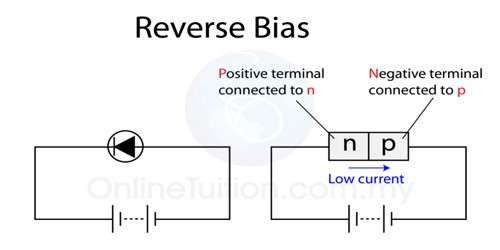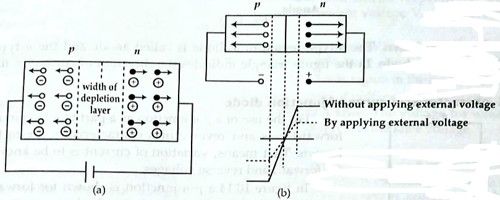Physics

# Reverse Bias working principle of Junction DiodeReverse Bias working principle of Junction Diode

If a p-type and n-type semiconductors are connected by a special technique then the junction surface is called a p-n junction. It is one of the simplest semiconductor devices around, and which has the characteristic of passing current in only one direction only. The process by which, a p-n junction diode blocks the electric current in the presence of applied voltage is called a reverse-biased p-n junction diode.

From external potential difference can be applied in the p-n junction in two ways, viz— forward bias and reverse bias. Here describe reverse-biased working principle.

Reverse Bias: In this case, an external voltage is applied in such a way as the height or width of the potential barrier increases. This type of bias is called reverse biasing. In order to apply reverse bias negative terminal of the battery is connected to the p-type end and positive terminal is connected to the n-type end. Due to the applied voltage electric field acts in favor of the electric field of the potential barrier.So the resultant electric field in the junction increases and the height of the potential barrier increases. In the figure, the application of reverse voltage and an increase of barrier height have been shown. As the potential barrier increases, so the resistance in the flow of charges increases and current does not flow in the circuit.

In Reverse bias, the battery attracts the electrons in the n-type and holes in the p-type and takes those away from the junction. So, the width of the junction increases and potential along the junction continuous to increase. The increase continues as long as the potential of the battery and the junction becomes equal.

When a reverse bias is applied, only a very small current flows through the junction. The reason for this current is that there are some holes and electrons in the n-type and p-type regions, respectively. The flow of those charges creates some small current. The value of this current is about a few μA. This current is called a reverse current.